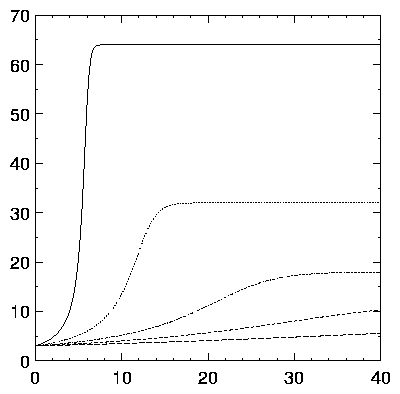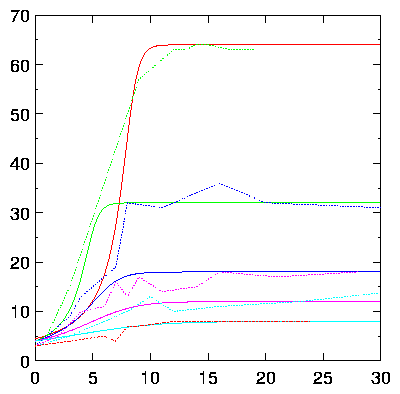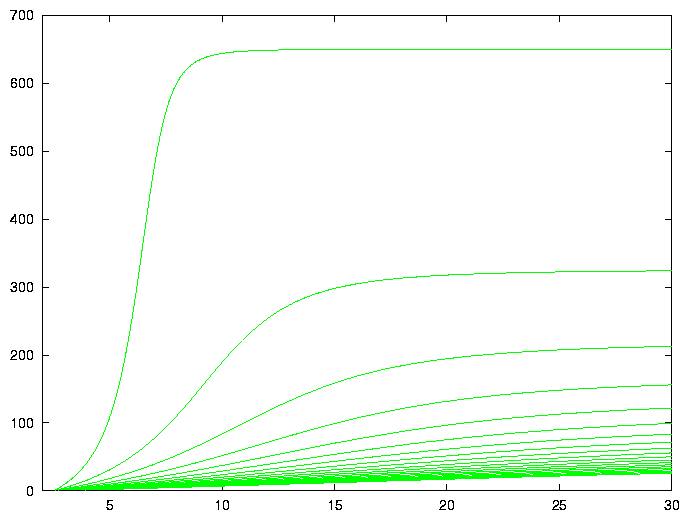Keith Briggshome · papers · thesis · talks · meetings · records · maths notes « · software · languages · music · travel · cv · students · maps · place-names · people · photos · links · ex libris · site map### W-ology, or, some exactly solvable growth models

by Keith Briggs (formerly at Department of Plant Sciences, University of Cambridge, Downing St., Cambridge CB2 3EA. Revised 1999 Nov 12; font adjustments 2005 July 11)

`To give full growth to that which still doth grow?' - Shakespeare, Sonnet CXV

#### Introduction

Aim of these notes: to show how some generalizations of logistic growth laws have elegant analytic solutions and speculate on their applications to modelling.

Lambert's W function is defined by

 W(x)exp(W(x)) = x,
or equivalently
 log(W(x))+W(x) = log(x).
Actually, W is multivalued, but I shall always use the principal branch, which satisfies W(0) = 0, and is defined on -e-1< x<∞.

#### Useful formulas

1. The solution of (a+bx)exp(cx)-d = 0 is
 x = W[cd/bexp(ac/b)]/c-a/b.
Example application: in New Phytol. 136, 362 (1997), the equation (q1+q2 R)exp(-q3R)-0.05 = 0 needed to be solved for the pathozone width R. This is trivial with the above formula: R = -W[-(q3/(20q2))exp(-q1q3/q2)]/q3-q1/q2. Similar transcendental algebraic equations arise in the computation of equilibria in graph-theoretical epidemic models.

2. W'(x) = W(x)/(x(1+W(x))) for x nonzero.
3. ∫ W(x)dx = x[W(x)-1+1/W(x)]+c

4. The inverse function W < -1 > is given by W < -1 > (x) = xexp(x).
5. The Taylor series of W around 0 is ∑k = 1[((-k)k-1)/k!]xk, and has radius of convergence e-1.

#### Differential equations

It is usually considered that the most general exactly solvable growth model is that of Richards:

 y'(t) = Β/ν y(1-(y/k)n)

(ν not 0, ν> -2) for which the solution is

 y(t) = Κ [1+J exp(- Β t)]-ν (2)

with J a function of Κ,ν and y(0).

Instead, I wish to consider models of the form

 y'(t) = yn (1-y)       n = 2,3,... .
The key to these are the integrals

1. ∫[dx/(x(1-bx))] = log(x)-log(bx-1)
2. ∫[dx/(x2(1-bx))] = b(log(x)-log(bx-1))-x-1
3. ∫integral[dx/(x3(1-bx))] = (-x-2/2-bx-1)-b2(log(bx-1)-log(x))

etc.

These allow us to give explicitly the solution of the initial value problem

 y'(t) = y2(1-y),       y(0) = y0
(3)
as

 y(t) = [1+W(ueu-t)]-1,       u = y0-1-1.
More generally,

 y'(t) = ay2(b-y),       y(0) = y0,   a > 0,   b > 0
(4)
has the solution

 y(t) = b[1+W(ueu-ab2 t)]-1,       u = b/y0-1.Figure 1: Solutions of equation (4) for y0 = 3,a = 0.001,b = 8,12,18,32,62

A very rough fit of equation (4) to some onion plant growth data is shown in Figure 2. No attempt has been made to optimize the parameters.Figure 2: Fit of equation (4) to onion growth data

Another differential equation, which can be interpreted as having a time-dependent growth rate, is

 v'(x) = v2(1-bv) x2(1-bx)
(5)
with the initial value v(0) = 0. Since this differential equation has a singular point at the origin, we need to add a condition such as v'(0) = 1 to pick out a solution of interest. The exact solution of equation (5) is explicitly given by

bv(x)-1 = [
[
[
[
 1+W[+exp{log(-b+1/x)+(1/x-c)/b-1}/b]
 (x < 1/b)
 1
 (x = 1/b)
 1+W[-exp{log(+b-1/x)+(1/x-c)/b-1}/b]
 (x > 1/b)
(6)
for c = constant. Some typical solutions are shown in Figure 3. These solutions tend to b[W(-exp(-c/b-1))+1]-1 as x tends to infinity.Figure 3: The functions v(x) for various b

#### Lotka-Volterra models

The two-species model

 dx dt
 =
 ax(1-y)
(7)
 dy dt
 =
 cy(1-x)
(8)
has solutions which are closed loops in the x-y plane. The exact solution (homework exercise) for the lower branch is

 y = -W[-Cxc/aexp(-cx/a)],       C = constant.

#### Take-home message

Don't assume that only solutions expressible with standard elementary transcendental functions are the only useful ones!

#### A numerical algorithm for W

```/* ANSI C code for W(x).  K M Briggs 98 Feb 12
http://www-epidem.plantsci.cam.ac.uk/~kbriggs/LambertW.c
Based on Halley iteration.  Converges rapidly for all valid x. */
double W(const double x) {
int i; double p,e,t,w,eps=4.0e-16; /* eps=desired precision */
if (x<-0.36787944117144232159552377016146086) {
fprintf(stderr,"x=%g is < -1/e, exiting.\n",x); exit(1); }
if (0.0==x) return 0.0;
/* get initial approximation for iteration... */
if (x<1.0) { /* series near 0 */
p=sqrt(2.0*(2.7182818284590452353602874713526625*x+1.0));
w=-1.0+p-p*p/3.0+11.0/72.0*p*p*p;
} else w=log(x); /* asymptotic */
if (x>3.0) w-=log(w);
for (i=0; i<20; i++) { /* Halley loop */
e=exp(w); t=w*e-x;
t/=e*(w+1.0)-0.5*(w+2.0)*t/(w+1.0); w-=t;
if (fabs(t)<eps*(1.0+fabs(w))) return w; /* rel-abs error */
} /* never gets here */
fprintf(stderr,"No convergence at x=%g\n",x); exit(1);
}
```

#### Bibliography

I've tried to make these notes self-contained, so all you should need is...

 Briggs, K. M. W-ology, or, some exactly solvable growth models

##### Footnotes:

1: Johann Heinrich Lambert: born 1728 Aug 26 in Mülhausen, Alsace; died 1777 Sep 25 in Berlin, Prussia. Lambert was a colleague of Euler and Lagrange at the Berlin Academy of Sciences. Amongst other achievements, he was the first to provide a rigorous proof that π is irrational.

This website uses no cookies. This page was last modified 2013-05-12 10:17 by.